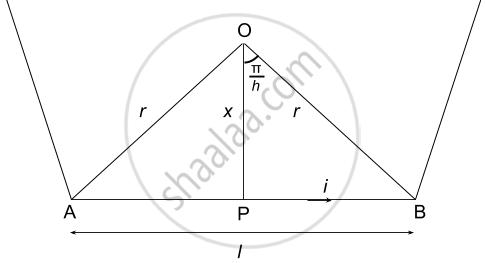Department of Pre-University Education, KarnatakaPUC Karnataka Science Class 12
Advertisement Remove all ads

# A Regular Polygon Of N Sides is Formed by Bending a Wire of Total Length 2πR Which Carries a Current I. (A) Find the Magnetic Filed B At the Centre of the Polygon. - Physics

Short Note

A regular polygon of n sides is formed by bending a wire of total length 2πr which carries a current i. (a) Find the magnetic filed B at the centre of the polygon. (b) By letting n → ∞, deduce the expression for the magnetic field at the centre of a circular current.

Advertisement Remove all ads

#### Solution(a)
Using figure,

$\text{ For a regure polygon of n - sides, the angle subtended at the centre is } \frac{2\pi}{n}$
$\tan\theta = \frac{l}{2x}$

$\Rightarrow x = \frac{l}{2\tan\theta}$

$\text{ considering angle to be small}$

$\frac{l}{2} = \frac{\pi r}{n}$

$\text{ Using Biot - Savart law for one side }$

$B = \frac{\mu_0}{4\pi}\frac{\text{idl }\sin\theta}{x^2}$

$\Rightarrow B = \frac{\mu_0}{4\pi}\frac{i(\sin\theta + \sin\theta)}{x^2} = \frac{\mu_0 i2\left( \tan\theta \right)\left( 2\sin\theta \right)}{4\pi l} \left( \text{ Putting value of r }\right)$

$\Rightarrow B = \frac{\mu_0 i2n\left( \tan\left( \frac{\pi}{n} \right) \right)\left( 2\sin\left( \frac{\pi}{n} \right) \right)}{4\pi\left( 2\pi r \right)} \left( \text{ Putting value of l } \right)$

$\text{ For n - sided polygon}$

$B' = nB$

$\Rightarrow B' = \frac{\mu_0 i n^2 \tan\left( \frac{\pi}{n} \right)\sin\left( \frac{\pi}{n} \right)}{2 \pi^2 r}$

(b)
when n→ ∞, polygon becomes a circle with radius r
and magnetic field will become  $B = \frac{\mu_0 i}{2r}$

Is there an error in this question or solution?
Advertisement Remove all ads

#### APPEARS IN

HC Verma Class 11, Class 12 Concepts of Physics Vol. 2
Chapter 13 Magnetic Field due to a Current
Q 24 | Page 251
Advertisement Remove all ads

#### Video TutorialsVIEW ALL 

Advertisement Remove all ads
Share
Notifications

View all notifications

Forgot password?